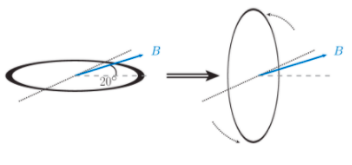# Problem: Joe has rolled a long electrical extension cord into a coil of radius rc =11 cm with Nc = 16 loops. The coil of extension cord is initially lying horizontally (flat) on the floor of Joe’s garage. Joe picks up the coil and rotates it 90° counterclockwise in tr= 0.85 seconds so that the coil is now vertically oriented (see figure)Part A. If the Earth’s magnetic field has strength of 0.50 gauss and makes an angle of 20.0 degrees with the garage floor, calculate the change in magnetic flux in Wb, through one of the loops of the coil during the rotationPart B. Calculate the average induced emf, in volts, in the coil during the rotationPart C. If the extension cord has a resistance of 3.0 ohms and formed a closed circuit without any other components, calculate the induced current, in amps, in the extension cord.

###### FREE Expert Solution

Magnetic flux:

$\overline{){{\mathbf{\varphi }}}_{{\mathbf{B}}}{\mathbf{=}}{\mathbf{B}}{\mathbf{A}}{\mathbf{c}}{\mathbf{o}}{\mathbf{s}}{\mathbf{\theta }}}$, where B is the magnetic field, A is the area perpendicular to the magnetic field, and θ is the angle between B and A.

Induced emf:

$\overline{){\mathbf{\epsilon }}{\mathbf{=}}{\mathbf{N}}\frac{\mathbf{∆}\mathbf{\varphi }}{\mathbf{∆}\mathbf{t}}}$, where N is the number of turns and t is the time of rotation.

Induced current:

$\overline{){{\mathbf{i}}}_{{\mathbf{c}}}{\mathbf{=}}\frac{\mathbf{\epsilon }}{\mathbf{R}}}$

80% (201 ratings)###### Problem Details

Joe has rolled a long electrical extension cord into a coil of radius rc =11 cm with Nc = 16 loops. The coil of extension cord is initially lying horizontally (flat) on the floor of Joe’s garage. Joe picks up the coil and rotates it 90° counterclockwise in tr= 0.85 seconds so that the coil is now vertically oriented (see figure)Part A. If the Earth’s magnetic field has strength of 0.50 gauss and makes an angle of 20.0 degrees with the garage floor, calculate the change in magnetic flux in Wb, through one of the loops of the coil during the rotation

Part B. Calculate the average induced emf, in volts, in the coil during the rotation

Part C. If the extension cord has a resistance of 3.0 ohms and formed a closed circuit without any other components, calculate the induced current, in amps, in the extension cord.

Frequently Asked Questions

What scientific concept do you need to know in order to solve this problem?

Our tutors have indicated that to solve this problem you will need to apply the Faraday's Law concept. You can view video lessons to learn Faraday's Law. Or if you need more Faraday's Law practice, you can also practice Faraday's Law practice problems.

What professor is this problem relevant for?

Based on our data, we think this problem is relevant for Professor Lee's class at HUNTER.